# Enthalpy, Entropy, And The Second Law of Thermodynamics

The objective is to develop the basics understanding of the following concepts:

• Internal Energy and First Law of Thermodynamics
• The cyclic and arbitrary process of a system
• Reversibility and Irreversibility
• Entropy and Enthalpy
• Second Law of Thermodynamics

## Internal Energy and First Law of Thermodynamics

When the energy of a molecule within a system is associated with the property of the system, then it is termed as Internal Energy(u).
Energy neither be created nor be destroyed and based on this principal system internal energy(u) changes whenever energy-crosses the system boundary.
Thus the first law of thermodynamics can be expressed as given below when heat/work interacts-with-the-system.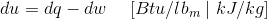In the above equation u is the internal energy per-unit-mass and q and w are heat and work per unit mass respectively. The sign convention adopted in the above equation is:
dq > 0 (considered as positive) ⇒ Heat transfer to the system
dq < 0 (considered as negative) ⇒ Heat transfer from the system dw > 0 (considered as positive) ⇒ work done by the system
dw < 0 (considered as negative) ⇒ work done on the system

### Cyclic and Arbitrary Process of a System

One of the important forms of the First law of thermodynamics is obtained when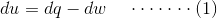We integration the above equation for a cyclic process.

A system said to be in cyclic process, when after undergoing random changes on account of heat/work returns to its original state.

Points to ponder are:

1. Integration of any state property differential is the difference of its limits.
2. Final state is same as the original state and there is no change in internal energy of the system.

Thus when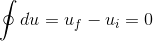Initial and final state of internal energy in the above equation is represented by i and f. Substituting above in equation (1) then,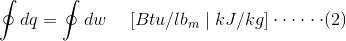Equation (2) is the representation of integral of all work done by the system or net work done by the system is equal to the integral of all heat transfer into the system. Engineering thermodynamics further explores the concepts of systems and processes.

### Arbitrary Process of a System

It is the outcome of the First law of thermodynamics and is related to equation (1) if a system involves an arbitrary process.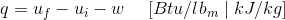In this equation q and w are the net heat transferred and net work for the process respectively, while uf and ui are final and initial values of internal energy(u). In a rigid and isolated adiabatic system (w = 0, q = 0), then its internal energy(u) remains unchanged. Then from eq(2) of a cyclic process.

### Reversibility and Irreversibility

A system is said to be undergoing a process when it initial state changes to final state. Properties like pressure, volume, enthalpy, temperature, entropy etc changes during a thermodynamic process. The second law of thermodynamics categories the processes under two heads

• Ideal or reversible processes
• Natural or irreversible processes

If temperature (t) and pressure (p) variations are infinitesimal in a system, which is undergoing-a-process, then the process can be termed as near equilibrium states or approaching reversibility.
The process is said to be reversible-internally if the original state is re-stored in reverse direction.
The process is said to be externally-reversible environment accompanying the change can also be reversed-in-sequence.
Reversible-process is one that is reversible both internally and externally.
In order to measure the success of a real processes, professionals uses reversible process as the measure for comparing and bringing the real and actual processes closer to reversibility by lowering down losses in order to increase the efficiency of the processes.

#### Irreversibility

When actual processes fails to meet the requirements of reversibility, then the processes is called irreversible.
In irreversibile process the initial state of the system and surrounding can’t be bring back to initial state from final state. Entropy of the system increases sharply in irreversible process and the value can’t be brought back to the initial value from the final value.
Irreversibility persists on account of variation in pressure, composition, temperature, composition main caused by heat transfer, friction in solid and liquid, chemical-reaction. Professions are busy in putting their efforts to bring down the effects of irreversibility in processes and mechanisms.

### Entropy and Enthalpy

Like internal energy, Entropy and Enthaly are thermodynamic properties. The entropy is represented by symbol s and change in entropy Δs in kJ/kg-K. Entropy is a state of disorder. Entropy is the subject of second Law of thermodynamics which describes entropy change in system and surrounding with respect to Universe.
Entropy is defined as ratio heat transfer to the absolute temperature in a system for a reversible thermodynamic path.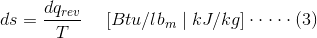Where, qrev denotes heat transfer along a reversible path.
Enthalpy (h) is the property of state and is defined as,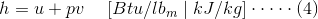Where, h is specific Enthalpy, u is specific internal energy, v is specific volume, p is the pressure.
From, equation (1)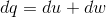Therefore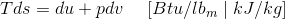By differentiating the eq (4) and substituting it in above equation, then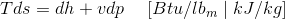Both of above equations are related to changes in entropy for reversible processes on account of changes in internal energy and volume in former and to change in enthalpy and pressure in later equation.
Since all quantities in these two equations are state properties, thus entropy is also a thermodynamic property.

## Second Law of Thermodynamics

Second law of thermodynamics is known for describing its limits on universe in terms of What universe can do. 2nd Law is more about dealing with inefficiencies, decay and degeneration.
We do activities in our day-to-day life which are by nature involves inefficient and irreversible processes.
2nd law of thermodynamics can be more conveniently expressed with respect to entropy:
Entropy defined as infinitesimal-change in entropy of a system (dS) is ratio of measured quantity of heat that has entered in to the closed system (dqrev) and the common temperature (T) at the point where the heat transfer took place.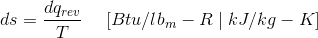Second law of thermodynamics states that “Entropy change is considered as non negative”.
OR
Universe energy is gradually moving towards the state of disorder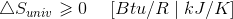Want To Learn Faster? 🎓
Get electrical articles delivered to your inbox every week.
No credit card required—it’s 100% free.